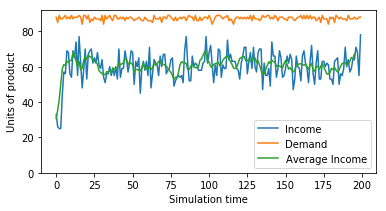# SOC Economic Model - Part 1

For more background on SOC and the model, see Self-Organized Criticality and Economic Applications

``````from matplotlib import pyplot as plt
import numpy as np
import os
import plotly
import plotly.plotly as py
import plotly.graph_objs as go
#from SOCEconModel import *

model_file_path = os.getcwd() + '/model.py'
model = SOCEconModel(1000,3,.4,.2,do_rewire=False,fix_demand=False)``````

## Variable setup for storing income and demand

Now let's set up some variables to store the income and demand information from the model run that we are interest in analyzing, and runs the model. This is done outside the model.py file because we want to separate the core model functionality from the analysis of model results. We might want to instantiate multiple models with different parameters, run them all, and track income and demand separately for each one.

We set up a list for income data, and one for demand data, and set the number of model steps. Each list entry will be a tally of income (or demand) for one model period or step. The for loop calls the model's step() function num_steps number of times, and after each step appends demand and income data to our lists:

For running the model outside a notebook, use model_run.py (which contains code similar to the above).

``````income, demand, num_steps = [], [], 200
for i in range(num_steps):
#model.period_income = 0
model.step()

income.append(model.period_income)
demand.append(model.period_demand)

income_long_avg = np.convolve(income, np.ones((int(num_steps/40),))/int(num_steps/40), mode='valid')
income_short_avg = np.convolve(income, np.ones((int(num_steps/200),))/int(num_steps/200), mode='valid')
demand_coverage = sum(income)/sum(demand)``````

## Plotting The Data - Basics

Now let's look at some plots of the income and demand data. We create a figure and some lines, add axis labels and set the y-axis limit to 0, and then display the plot. The if statement gives us two sets of lines, one for short runs (< 5000 steps) and one for longer runs - this is because for longer runs, the noise in income will look messy when plotted.

This version of the plot uses matplotlib.

``````#matplotlib version
fig = plt.figure(1,figsize=(6,3))
long_steps = 5000

if num_steps <= long_steps:
income_line, = plt.plot(income, label='Income')
demand_line, = plt.plot(demand, label='Demand')
income_avg_line, = plt.plot(income_long_avg, label='Income average')
plt.legend([income_line, demand_line,income_avg_line],
['Income', 'Demand', 'Average Income'],loc='best')
else:
income_short_line, = plt.plot(income_short_avg, label='Line 2')
demand_line, = plt.plot(demand, label='Line 1')
income_long_line, = plt.plot(income_long_avg, label='Line 1')
plt.legend([income_short_line, demand_line,income_long_line],
['Income (short avg.)', 'Demand', 'Income (long avg.)'],loc='best')

plt.xlabel('Simulation time')
plt.ylabel('Units of product')
plt.ylim(bottom=0)
plt.show()``````# Using Plotly

Here's some code and a similar plot, this time using Plotly instead of matplotlib. Dash and Plotly make is easy to build beautiful web-friendly applications using interactive data visualization. Play around with the plot below - you can zoom, select an area to view in detail, turn lines on and off by clicking in the legend, and more. This plot is visually more appealing, and it's more useful. What's really cool is that whenever you create plots in your code, Plotly automatically saves them to your account for later use. This was originally generated in a Jupyter notebook, but now is available in my saved plots at the plotly site. All I had to do was include a small code snippet (see below).

``````#plotly version
scatter_x = list(range(num_steps))

trace0 = go.Scatter(
x = scatter_x,
y = income,
mode = 'lines',
name = 'Income'
)

trace1 = go.Scatter(
x = scatter_x,
y = income_short_avg,
mode = 'lines',
name = 'Income (short avg)'
)

trace2 = go.Scatter(
x = scatter_x,
y = income_long_avg,
mode = 'lines',
name = 'Income (long avg)'
)

trace3 = go.Scatter(
x = scatter_x,
y = demand,
mode = 'lines',
name = 'Demand'
)

layout = go.Layout(
autosize=True,
width=600,
height=400,
yaxis=dict(
range=[0, max(max(income), max(demand))])
)

if num_steps <= long_steps:
data = [trace0, trace2, trace3]
else:
data = [trace1, trace2, trace3]

fig = go.Figure(data=data, layout=layout)
py.iplot(fig, filename='SOCModel-basic')``````

Here's the embed code:

``````<div>
<a href="/ https://plot.ly/ ~gdcutting/16/?share_key=elPbdRQxSRt5OdiUEdZWZu" target="_blank" title="SOCModel-basic" style="display: block; text-align: center;"><img src="/ https://plot.ly/ ~gdcutting/16.png?share_key=elPbdRQxSRt5OdiUEdZWZu" alt="SOCModel-basic" style="max-width: 100%;width: 600px;" width="600" onerror="this.onerror=null;this.src=' https://plot.ly/404.png ';" /></a>
<script data-plotly="gdcutting:16" sharekey-plotly="elPbdRQxSRt5OdiUEdZWZu" src="/ https://plot.ly/embed.js " async></script>
</div>``````